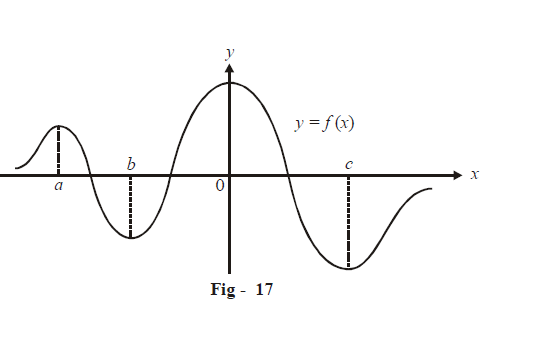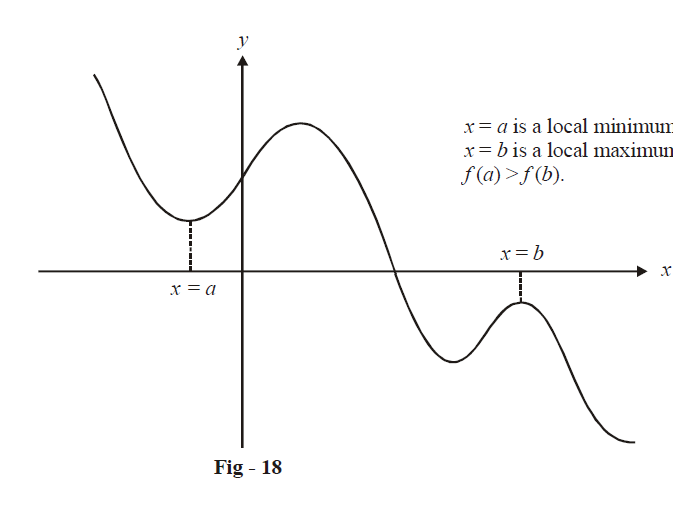# Introduction to Maxima and Minima

Although the name itself is suggestive, we introduce the concept of maxima and minima here through a simple example:Consider an arbitrary function f(x).

The concept of Maxima and Minima is a way to characterize the peaks and troughs of f(x). For example, we see that there is a peak at $$x~=~a;$$ this point is therefore a local maximum; similarly, $$x=\text{ }0$$ is also a local maximum; however, since f(0) has the largest value on the entire domain, $$x=\text{ }0$$ is also a global maximum.

Analogously, $$x = b$$ and $$x = c$$ are local minimum points; $$x = c$$ is also a global minimum.

Having introduced the concept intuitively, we can now introduce more rigorous definitions:

 (A) LOCAL MAXIMUM: A point $$x = a$$ is a local maximum for f(x) if in the neighbourhood of a i.e in $$\left( {a - \delta ,a + \delta } \right)$$ where $$\delta$$ can be made arbitrarily small, for $$f\left( x \right) < f\left( a \right)$$ for all $$x \in \left( {a - \delta ,a + \delta } \right)\backslash \left\{ a \right\}$$. This simply means that if we consider a small region (interval) around $$x = a,$$ f(a) should be the maximum in that interval. (B) GLOBAL MAXIMUM: A point $$x = a$$ is a global maximum for f(x) if $$f\left( x \right) \ge f\left( a \right)$$ for all $$x \in D$$ (the domain of f(x)). (C) LOCAL MINIMUM: A point $$x = a$$ is a local minimum for f(x) if in the neighbourhood of a, i.e. in $$\left( {a - \delta ,a + \delta } \right),$$ (where $$\delta$$ can have arbitrarily small values),$$f\left( x \right) > f\left( a \right)$$ for all$$x \in \left( {a - \delta ,a + \delta } \right)\backslash \left\{ a \right\}.$$ This means that if we consider a small interval around $$x=a,f\left( a \right)$$ should be the minimum in that interval. (D) GLOBAL MINIMUM: A point $$x = a$$ is a global minimum for f(x) if $$f\left( x \right) \ge f\left( a \right)$$ for all $$x \in D$$ (the domain of f(x)).

As examples, $$f\left( x \right) = \left| x \right|$$  has a local (and global) minimum at $$x = 0,$$ $$f(x) = {x^2}$$ has a local (and global) minimum at $$x=\text{}0,f\left( x \right)\text{}=\text{}sinx$$ has local (and global) maxima at \begin{align}x = 2n\pi + \frac{\pi }{2},n \in \mathbb{Z}\end{align}  and local (and also global) minima at \begin{align}x = 2n\pi - \frac{\pi }{2},n \in \mathbb{Z}\end{align}. Note that, for a function $$f\left( x \right),$$ a local minimum could actually be larger than a local maximum elsewhere. There is no restriction to this. A local minimum value impliese a minimum only in the immediate ‘surroundings’ or ‘neighbourhood’ and not ‘globally’; similar is the case for a local maximum point.To proceed further, we now restrict our attention only to continuous and differentiable functions.